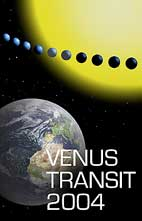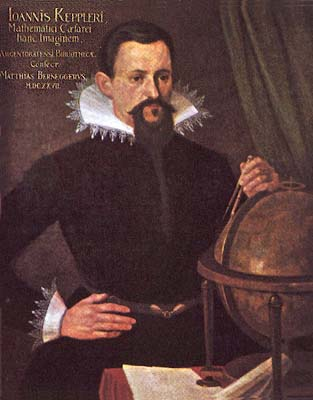Appendix 2

# Kepler's Third Law

Kepler's Third Law states that the semi-major axes av, ae of the elliptical orbits of Venus and the Earth and their orbital periods T v , T e satisfy the following relationship:
(a e / a v ) 3 = (T e / T v 2 )

If we assume circular orbits for Venus and the Earth, Kepler's Third Law says that their radii r v , r e and their orbital periods Tv, Te satisfy the following relationship:
(r e / r v ) 3 = (T e / T v ) 2Johannes Kepler used Tycho Brahe's observations to formulate his laws

Back to the EduSheet 3